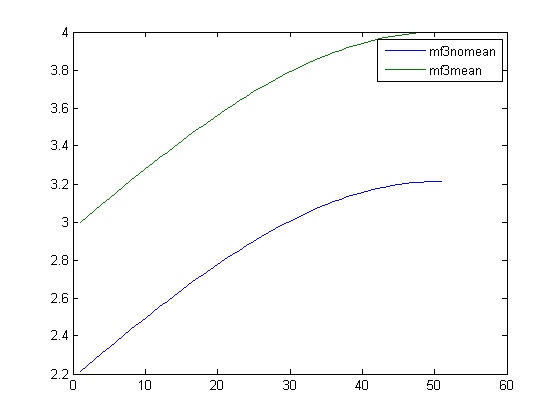# Combining Functions

For a lot of calculations, it makes sense to combine operations into a single expression. You can sometimes do this easily when you have function handles and you generate one final function that you can apply.

### Function Composition

Suppose you want to call g(f(x)), where the output of the function f is the input for the function g.

f = @(x) sin(x);
g = @(x) cos(x);
fCompg = @(x) g(f(x));

% Test the composition.

x = 0:pi/100:pi/2;
fx = f(x);
gfx = g(fx);
gfx2 = fCompg(x);
gfsEqual = isequal(gfx,gfx2)
gfsEqual =
1


### Other Combinations.

Suppose instead you want to add some functions together under certain circumstances. Sometimes, but not always. It's messy if you don't commit to evaluating a function handle with a fixed name. So, here's a pattern you can use to achieve this effect.

myfunc = @(x) sin(x);
mn = @(x) mean(x);
three = @(x) 3+zeros(size(x));
subtractMean = false;
add3 = true;

Suppose I want to add a constant offset only.

if subtractMean
myfunc = @(x) bsxfun(@minus, myfunc(x), mn(x));
end
myfunc = @(x) myfunc(x) + three(x);
end

Try this on the data.

mf3mean = myfunc(x);

Now, regardless of which functions we combine with the original function, we have a function handle to evaluate. You can use this to allow users to specify additional information as you build up an appropriate function for your calculation.

Let's try a different combination. Let's suppose I want the mean value to be around 3. I can first remove the mean and then add the offset.

myfunc = @(x) sin(x);
subtractMean = true;
if subtractMean
myfunc = @(x) bsxfun(@minus, myfunc(x), mn(x));
end
myfunc = @(x) myfunc(x) + three(x);
end
mf3nomean = myfunc(x);

Notice the order of operations matters here. If I removed the mean after adding the constant, the final mean should be 0. If I remove it before adding the offset, I have no reason to expect that.

mean(mf3nomean)
mean(mf3mean)
ans =
2.8485
ans =
3.6339

h = plot(mf3nomean);
hold all
h(2) = plot(mf3mean);
hold off
legend(h,{'mf3nomean', 'mf3mean'})### Do You Combine Functions?

Do you have situations in which you conditionally apply functions? Would this technique help you build up the functions you want more easily? Let me know here.

Published with MATLAB® 7.10

|